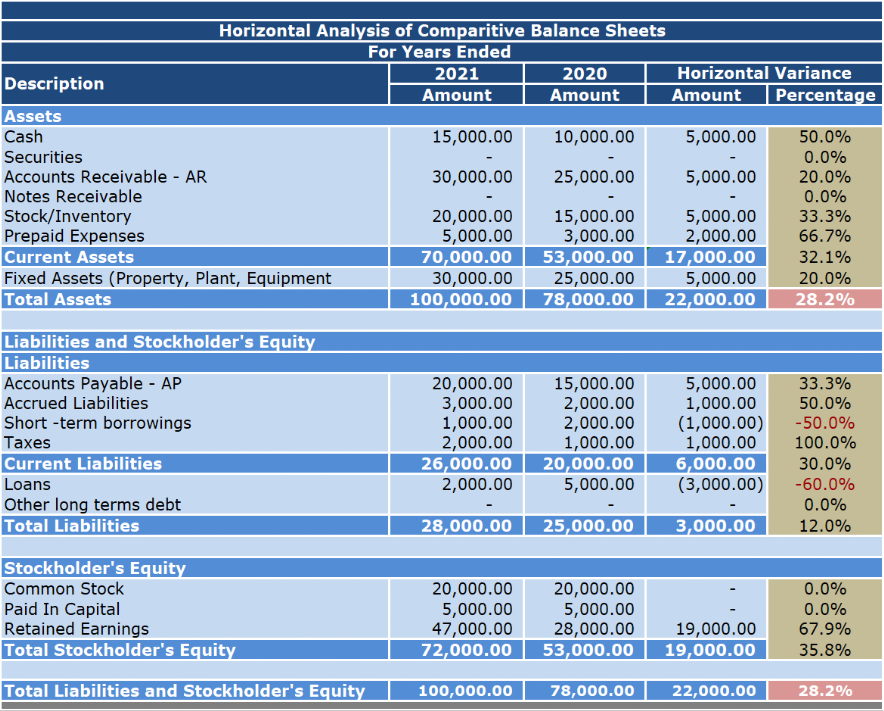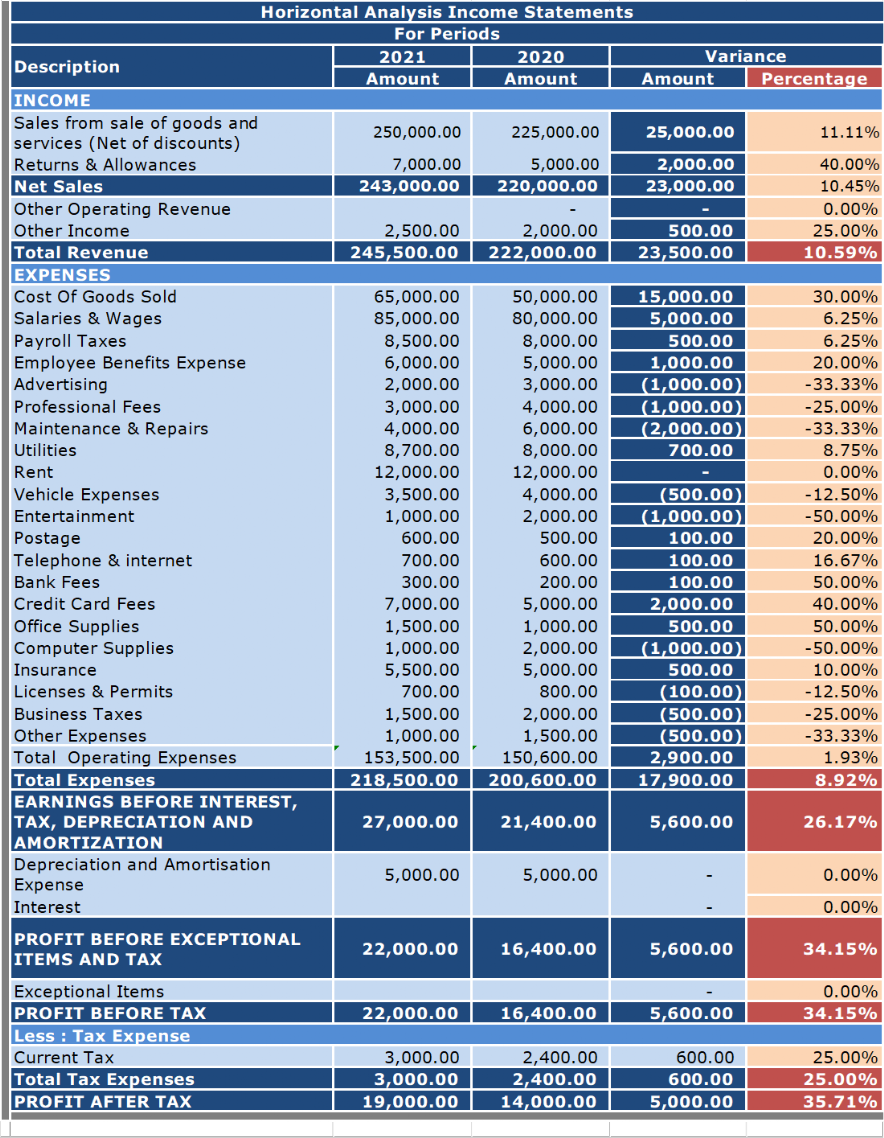# Horizontal Analysis - BC Bookkeeping Tutorials|dwmbeancounter.comTitle
Go to content

## Horizontal Analysis

Horizontal- Vertical AnalysisWhat is Horizontal Analysis ?
Horizontal analysis is a financial statement analysis method that presents changes in the amounts of financial statement items over a period of time. The analysis uses two or more periods. Periods can be a month, a quarter, or a year. The analysis aids you in detecting patterns and trends and identifying any areas that may need further investigation and attention. Horizontal analysis of financial statements can be performed on any of the items in the income statement, balance sheet, and cash flow statement.

The changes are generally shown both in dollar amounts and percentage amounts.

Changes are measured against a base year(s) using the following formulas:
Percentage Change = (Current period amount — Base period amount)  / Base period amount X 100
Percentage differences in certain items are compared over a period of time. The absolute currency amounts are converted into the percentages for the purpose of comparison.This method is useful when comparing performance of two companies of different scale and size.

Dollar Amount Change =Current period amount - Base period amount
This method is helpful in identifying the items which are changing the most.

Sample Horizontal Analysis of a Balance SheetExample of Calculations

We'll use Cash for our example.

Dollar Amount Change=Current period amount - Base period amount
Dollar Amount Change of Cash=15,000 - 10,000
Dollar Amount Change of Cash=5,000

Percentage Change = (Current period amount — Base period amount)  / Base period amount X 100
Percentage Change of Cash = (15,000 — 10,000 ) / 10,000 X 100
Percentage Change of Cash = 50%
Sample Horizontal Analysis Of an Income StatementExample of Calculations

We'll use Cost Of Goods Sold for our example.

Dollar Amount Change=Current period amount - Base period amount
Dollar Amount Change of Cost Of Goods Sold =65,000 - 50,000
Dollar Amount Change of Cost Of Goods Sold=15,000

Percentage Change = (Current period amount — Base period amount)  / Base period amount X 100
Percentage Change of Cost Of Goods Sold = (65,000 — 50,000 ) / 50,000 X 100
Percentage Change of Cost Of Goods Sold = 30%
Both these statements determine the dollar amount of change and the percentage amount of change for two or more periods.

Note: We could have even been a little fancier by grouping our expenses into categories such as Selling Expenses, and General & Administrative Expenses in our Income Statement. We could also expand the number of periods that we evaluated.

Now that we have the amounts what's next. That's half the battle, the other half is reviewing and discussing these statements with your bookkeeper or accountant if you have one. If not, you need to undertake this task yourself.Some General Guidelines on How to Analyze the Amounts
From period to period, some items increase while others decrease so did we do well or not so well ? Many of the figures are related to our level of sales (sales volume). Some expenses remain the same (fixed expenses), while others increase or decrease depending (variable expenses) on the level of sales.

Variable costs fluctuate depending on how many products you produce or services you provide. When sales are high, variable costs increase. When sales are low, variable costs decrease. Some example of variable costs include commission on sales, credit card fees, direct labor, direct material, and production supplies.

Unlike variable costs, fixed costs are not affected by sales. No matter how many products you produce or services you provide, your fixed costs do not change. Fixed costs are unavoidable, basic operating expenses that your business pays. Since you pay the same amount from month to month, fixed costs are considered periodic expenses. Common examples of fixed costs include management salaries, rental and lease expenses, salaries, insurance expense, property taxes, interest expenses, depreciation, and some utilities and advertising expenses.

Key Figures:

Balance Sheet Items
Cash Position
Increase/Decrease Inventory
Increase/Decrease in Accounts Receivable
Increase/Decrease in Accounts Payable
Current Assets
Current Liabilities
Total Owner's Equity

Income Statement Items
Net Income/Profit
Increase/Decrease Sales
Increase/Decrease Costs & Expenses
Fixed Costs
Variable Costs
What's Vertical Analysis ?
Back to content